# Colleges with the lowest SAT scores in Oregon

Top 10 colleges in Oregon with the lowest SAT scores
Looking for the colleges with the lowest SAT scores in Oregon? Well you're in luck! We've compiled a national college database and have created a list of the top 10 universities with the lowest SAT scores in Oregon below. If you are not a good test taker or worried about your test scores, this list is for you. These are the schools whose applicants had the lowest average SAT scores in Oregon, which means that you can get into these colleges with a lower SAT score. We also include each college's ACT scores and acceptance rate so that you can see where you would have the easiest time getting in. Read on to find out more.

## Warner Pacific College SAT scores

The average SAT score for Warner Pacific College is 990.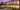The average SAT score of 990 breaks down into:

• SAT math: 480

The average ACT score for Warner Pacific College is 18 and their acceptance rate is 62.8%.

## Western Oregon University SAT scores

The average SAT score for Western Oregon University is 1040.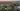The average SAT score of 1040 breaks down into:

• SAT math: 510

The average ACT score for Western Oregon University is 20 and their acceptance rate is 80.6%.

## Eastern Oregon University SAT scores

The average SAT score for Eastern Oregon University is 1042.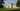The average SAT score of 1042 breaks down into:

• SAT math: 520

The average ACT score for Eastern Oregon University is 20 and their acceptance rate is 98.4%.

## Concordia University Portland Oregon SAT scores

The average SAT score for Concordia University Portland Oregon is 1070.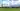The average SAT score of 1070 breaks down into:

• SAT math: 530

The average ACT score for Concordia University Portland Oregon is 22 and their acceptance rate is 53.8%.

## Southern Oregon University SAT scores

The average SAT score for Southern Oregon University is 1100.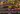The average SAT score of 1100 breaks down into:

• SAT math: 530

The average ACT score for Southern Oregon University is 22 and their acceptance rate is 78.2%.

## Linfield College SAT scores

The average SAT score for Linfield College is 1110.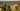The average SAT score of 1110 breaks down into:

• SAT math: 550

The average ACT score for Linfield College is 23 and their acceptance rate is 81%.

## Portland State University SAT scores

The average SAT score for Portland State University is 1119.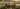The average SAT score of 1119 breaks down into:

• SAT math: 550

The average ACT score for Portland State University is 22 and their acceptance rate is 90%.

## George Fox University SAT scores

The average SAT score for George Fox University is 1150.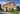The average SAT score of 1150 breaks down into:

• SAT math: 560

The average ACT score for George Fox University is 23 and their acceptance rate is 78.3%.

## Pacific University Oregon SAT scores

The average SAT score for Pacific University Oregon is 1150.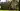The average SAT score of 1150 breaks down into:

• SAT math: 570

The average ACT score for Pacific University Oregon is 24 and their acceptance rate is 79%.

## University of Oregon SAT scores

The average SAT score for University of Oregon is 1185.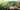The average SAT score of 1185 breaks down into:

• SAT math: 585